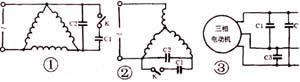# 介紹幾種簡便易行的將380v電機改220v電機的方法

C1=1950I/U×cosθ

C2=(1～4)C1

C2=(2～4)C1

C3=2C1

R=0.25U/IC=(Ssin(60+φ)×10^6)/(1.5WU^2)

L=(1.5U^2)/(WSsin(60-φ))